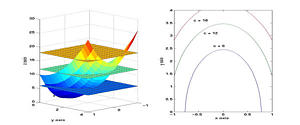# Jeffery Cooper

Professor Emeritus
Mathematics Department
The University of Maryland
College Park, MD 20742

email: jec@math.umd.edu

## Some notes for Multivariable Calculus

### Acceleration and curvature

These notes (10 pages) give a more complete treatement of acceleration and curvature. The notes are in pdf. Click here .

### Curl and divergence, and the theorems of Green, Gauss and Stokes.

These notes (28 pages) are written to give a better understanding of these important ideas.

## MATLAB materials

### MATLAB HELP

For help with computers, MATLAB, Mathematica, etc, this link will take you to the computer help page of the Mathematics Department. Computer Resources

### Introduction to MATLAB

(exerpted from A MATLAB Companion for Multivariable Calculus, Harcourt/Academic Press, 2001)

Here is a short introduction to MATLAB that can be downloaded in either pdf or postscript format.

# Books

## Working Analysis

A text for a two semester course in advanced calculus that gives rigorous treatment of the basic concepts of analysis, and then uses them to solve problems. There are many opportunities for computation as well.

MATLAB codes for Working Analysis

Errata in pdf## Introduction to Partial Differential Equations with MATLAB

### Birkhauser (1998).

This is a text for the standard Junior-Senior year introductory course for partial differential equations. There is an extensive computer component, using MATLAB. In addition to the usual separation of variables, we also introduce elementary numerical methods for each equation, and treat some nonlinear problems.

### PDE Gallery

Here are some examples of graphics generated by the MATLAB codes.
Formation of shock wave
The Dirichlet problem.
Solution of the heat equation.
Nonlinear string equation

### Errata for the first printing

For a list of text errata in postscript format, click here: Errata